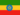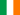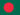# Transformações do Log

• Nota de Estudos
• Rever TópicosHenock A. 0 0 doneRachel N. 0 0 Log transformationJahidul I. 0 0 Logarithms are one of the most important mathematical tools in the toolkit of statistical modeling, so you need to be very familiar with their properties and uses. A logarithm function is defined with respect to a “base”, which is a positive number: if b denotes the base number, then the base-b logarithm of X is, by definition, the number Y such that bY = X. For example, the base-2 logarithm of 8 is equal to 3, because 23 = 8, and the base-10 logarithm of 100 is 2, because 102 = 100. There are three kinds of logarithms in standard use: the base-2 logarithm (predominantly used in computer science and music theory), the base-10 logarithm (predominantly used in engineering), and the natural logarithm (predominantly used in mathematics and physics and in economics and business). In the natural log function, the base number is the transcendental number “e” whose deciminal expansion is 2.718282…, so the natural log function and the exponential function (ex) are inverses of each other. The only differences between these three logarithm functions are multiplicative scaling factors, so logically they are equivalent for purposes of modeling, but the choice of base is important for reasons of convenience and convention, according to the setting.Jahidul I. 0 0 what is logarithm ?
• Text Version### Você recebeu uma nova notificação

Clique aqui para visualizar todos eles